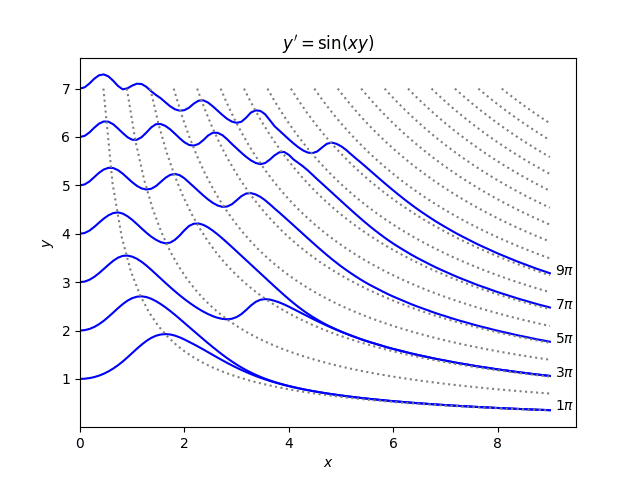# Ripples and hyperbolas

I ran across a paper  this morning on the differential equation

y‘ = sin(xy).

The authors recommend having students explore numerical solutions to this equation and discover theorems about its solutions.Their paper gives numerous theorems relating solutions and the hyperbolas xy = a: how many times a solution crosses a hyperbola, at what angle, under what conditions a solution can be tangent to a hyperbola, etc.

The plot above is based on a plot in the original paper, but easier to read. It wasn’t so easy to make nice plots 40 years ago. In the original plot the solutions and the asymptotes were plotted with the same thickness and color, making them hard to tell apart.

## More differential equation posts

 Wendell Mills, Boris Weisfeiler and Allan M. Krall. Discovering Theorems with a Computer: The Case of y‘ = sin(xy). The American Mathematical Monthly, Nov., 1979, Vol. 86, No. 9 (Nov., 1979), pp. 733-739

## One thought on “Ripples and hyperbolas”

1. Hi John,

You might find Carl Bender’s course on Perturbation Theory interesting, in case you haven’t heard of it. In particular, in his last class (link below, t=1:06:00) he talks about this particular equation (well, with cos instead of sin) and has a really nice an elegant explanation for how it behaves, and why it converges to every other isoline of pi in your posted graph, instead to every isoline, and so on. He really is an excellent teacher.

https://youtu.be/RqZ0VsEc4F0

Best,